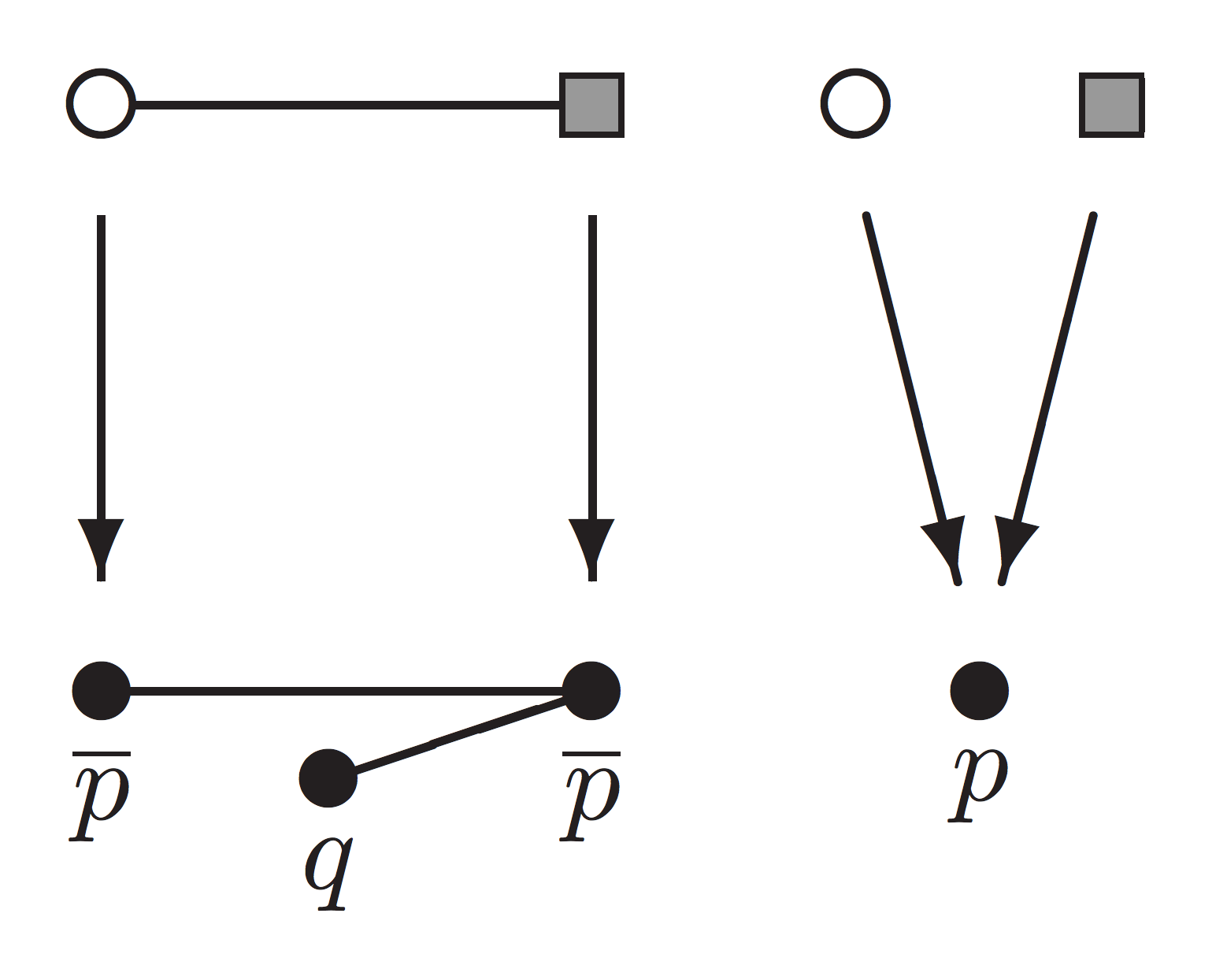### Dominic Hughes - Selected Papers

Proofs without syntax [pdf - arxiv draft - bibtex]
Annals of Mathematics 143(3), 1065-1076, Nov 2006.

Abstract
``Mathematicians care no more for logic than logicians for mathematics.'' [Augustus de Morgan, 1868]

Proofs are traditionally syntactic, inductively generated objects. This paper presents an abstract mathematical formulation of propositional calculus (propositional logic) in which proofs are combinatorial (graph-theoretic), rather than syntactic. It defines a combinatorial proof of a proposition ϕ as a graph homomorphism h : C -> G(ϕ), where G(ϕ) is a graph associated with ϕ, and C is a coloured graph. The main theorem is soundness and completeness: ϕ is true iff there exists a combinatorial proof h : C -> G(ϕ).